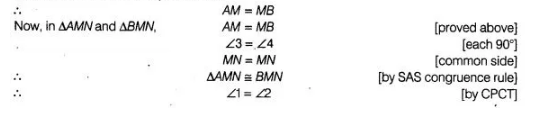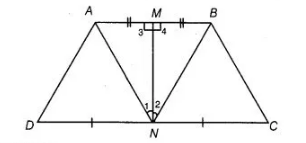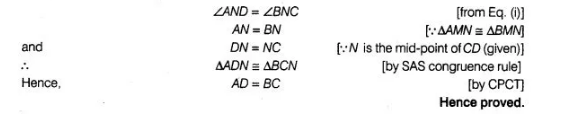# Line segment joining the mid-points`
Question:

Line segment joining the mid-points M and N of parallel sides AB and DC, respectively of a trapezium ABCD is perpendicular to both the sides AB and DC. Prove that AD = BC.

Solution:

Given In trapezium $A B C D$, points $M$ and $N$ are the mid-points of parallel sides $A B$ and $D C$ respectively and join $M N$, which is perpendicular to $A B$ and $D C$.

To prove

$A D=B C$

Proof Since, $M$ is the mid-point of $A B$.On multiplying both sides of above equation by $-1$ and than adding $90^{\circ}$ both sides, we get

$90^{\circ}-\angle 1=90^{\circ}-\angle 2$

$\Rightarrow \quad \angle A N D=\angle B N C$ ...(i)Now, in $\triangle A D N$ and $\triangle B C N$,